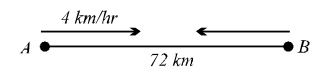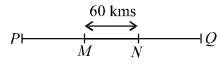## Speed, Time and Distance

#### Speed, Time and Distance

1. A man is standing on a railway bridge which is 50 metres long. He finds that a train crosses the bridge
 in 4 1 seconds but himself in 2 seconds. 2
Find the length of the train and its speed.
1. 60 m ; 20 m/sec
2. 40 m ; 20 kmph
3. 40 m ; 20 m/sec
4. 40 m ; 25 m/sec

1. Let the length of the train be x metres
Then, the time taken by the train to cover (x + 50) metres is

 4 1 seconds 2

 = x + 50 9 2

 or 2x + 100 m per second   ...(i) 9

Again, the time taken by the train to cover x metres in 2 seconds.
 ∴  Speed of the train = x metre per second   ...(ii) 2

From equations (i) and (ii), we have
 2x + 100 = x 9 2

⇒  4x + 200 = 9x
⇒  5x = 200
⇒  x = 40
∴  Length of the train = 40 metres
∴  Speed of the train
 = x = 40 = 20 m per sec. 2 2

##### Correct Option: C

Let the length of the train be x metres
Then, the time taken by the train to cover (x + 50) metres is

 4 1 seconds 2

 = x + 50 9 2

 or 2x + 100 m per second   ...(i) 9

Again, the time taken by the train to cover x metres in 2 seconds.
 ∴  Speed of the train = x metre per second   ...(ii) 2

From equations (i) and (ii), we have
 2x + 100 = x 9 2

⇒  4x + 200 = 9x
⇒  5x = 200
⇒  x = 40
∴  Length of the train = 40 metres
∴  Speed of the train
 = x = 40 = 20 m per sec. 2 2

1. Two men set out the same time to walk towards each other from two points A and B, 72 km apart. The first man walks at the rate of 4 km per hr. The second man walks 2 km in the first hour,
 2 1 km in the second hour, 3 km in the third hour and so on. 2
Find the time after which the two men will meet.
1. 8 hours
2. 9 hours
3. 8.5 hours
4. 9.5 hours

1. Let the two men meet after t hours.Distance covered by the first man starting from A = 4 t km.
Distance covered by the second man starting from B

 = 2 + 2.5 + 3 +......+2 +t − 12

This is an arithmetic series of t terms with 1/2 as common difference.
∴  By applying formula
 S = n2a + (n − 1)d2

Where, n = no. of terms
a = first term
d = common difference
We have its sum
 S = t(2 × 2) + (t − 1) × 12 2

 = 2t + t2 − t 4

Total distance covered by two men =
 = 4t + 2t + t2 − t = 72 4

or
 = 6t + t2 − t = 72 4

or  24t + t2 – t = 288
or  t2 + 23t – 288 = 0
or  t2 – 9t + 32t – 288 = 0
or  t (t–9) + 32 (t – 9) = 0
or  (t – 9) (t + 32) = 0
∴  Either t – 9 = 0 ⇒ t = 9
or  (t + 32) = 0 ⇒ t = – 32
Time cannot be negative.
Hence, the two men will meet after 9 hrs.

##### Correct Option: B

Let the two men meet after t hours.Distance covered by the first man starting from A = 4 t km.
Distance covered by the second man starting from B

 = 2 + 2.5 + 3 +......+2 +t − 12

This is an arithmetic series of t terms with 1/2 as common difference.
∴  By applying formula
 S = n2a + (n − 1)d2

Where, n = no. of terms
a = first term
d = common difference
We have its sum
 S = t(2 × 2) + (t − 1) × 12 2

 = 2t + t2 − t 4

Total distance covered by two men =
 = 4t + 2t + t2 − t = 72 4

or
 = 6t + t2 − t = 72 4

or  24t + t2 – t = 288
or  t2 + 23t – 288 = 0
or  t2 – 9t + 32t – 288 = 0
or  t (t–9) + 32 (t – 9) = 0
or  (t – 9) (t + 32) = 0
∴  Either t – 9 = 0 ⇒ t = 9
or  (t + 32) = 0 ⇒ t = – 32
Time cannot be negative.
Hence, the two men will meet after 9 hrs.

1. Two boys begin together to write out a booklet containing 817 lines. The first boy starts with the first line, writing at the rate of 200 lines an hour and the second boy starts with the last lines then writes line 816 and so on. Backward proceeding at the rate of 150 lines an hour. At what line will they meet?
1. 467th line
2. 468th line
3. 470th line
4. 475th line

1. Writing ratio = 200 : 150 = 4 : 3
In a given time first boy will be writing the line number

 4 × 817 7

 = 3268 th line = 466 6 th line 7 7

or, 467 th line
Hence, both of them shall meet on 467th line.

##### Correct Option: A

Writing ratio = 200 : 150 = 4 : 3
In a given time first boy will be writing the line number

 4 × 817 7

 = 3268 th line = 466 6 th line 7 7

or, 467 th line
Hence, both of them shall meet on 467th line.

1. Two trains A and B are 110 km apart on a straight line. One train starts from A at 7 a.m. and travels towards B at 20 km per hr. Another train starts from B at 8 am. and travels towards A at a speed of 25 km per hr. At what time will they meet?
1. 10 : 15 a.m.
2. 09 : 50 a.m.
3. 09 : 30 a.m.
4. 10 : 00 a.m.

1. Let they meet x hrs after 7 am.
Distance covered by A in x hours = 20x km
Distance covered by B in (x –1) hr.
= 25 (x – 1) km
∴  20x + 25 (x – 1) = 110
⇒  20x + 25x – 25 = 110
⇒  45x = 110 + 25 = 135
⇒  x = 3
∴  Trains meet at 10 a.m.

##### Correct Option: D

Let they meet x hrs after 7 am.
Distance covered by A in x hours = 20x km
Distance covered by B in (x –1) hr.
= 25 (x – 1) km
∴  20x + 25 (x – 1) = 110
⇒  20x + 25x – 25 = 110
⇒  45x = 110 + 25 = 135
⇒  x = 3
∴  Trains meet at 10 a.m.

1. A train after travelling 100 kms from P meets with an accident and then proceeds
 at 3 th of its original speed and arrives 4
at the terminus Q 90 minutes late. Had the accident occurred 60 kms further on, it would have reached 15 minutes sooner. Find the original speed of the train and the distance PQ.
1. 65 kmph; 480 km
2. 75 kmph; 450 km
3. 80 kmph; 460 km
4. 85 kmph; 460 km

1.Let P be the starting point, Q the terminus, M and N the places where accidents occur.
At (3/4)th of the original speed, the train will take 4/3 of its usual time to cover the same distance i.e., (1/3)rd more than the usual time.
(1/3)rd of the usual time to travel a distance of 60 kms between MN = 15 min.
∴  Usual time to travel 60 kms

 = 15 × 3 = 45 min. = 3 hr. 4

∴  Usual speed of the train per hour
 = 60 × 4 = 80 km per hr. 3

Usual time taken to travel MQ = 90 × 3
 = 270 min. or 9 hrs. 2

 = 80 × 9 = 360 km. 2

Therefore, the total distance PQ
= PM + MQ
= 100 + 360 = 460 kms.

##### Correct Option: CLet P be the starting point, Q the terminus, M and N the places where accidents occur.
At (3/4)th of the original speed, the train will take 4/3 of its usual time to cover the same distance i.e., (1/3)rd more than the usual time.
(1/3)rd of the usual time to travel a distance of 60 kms between MN = 15 min.
∴  Usual time to travel 60 kms

 = 15 × 3 = 45 min. = 3 hr. 4

∴  Usual speed of the train per hour
 = 60 × 4 = 80 km per hr. 3

Usual time taken to travel MQ = 90 × 3
 = 270 min. or 9 hrs. 2

 = 80 × 9 = 360 km. 2

Therefore, the total distance PQ
= PM + MQ
= 100 + 360 = 460 kms.